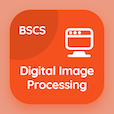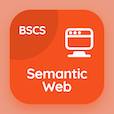Online CS Degree Courses

Digital Logic Design Quizzes

Digital Logic Design Quiz - Complete

Study NAND Implementation Multiple Choice Questions and Answers (MCQs), nand implementation quiz answers PDF, test 35 to download Digital Logic Design book. Simplification of Boolean Functions MCQ trivia questions, nand implementation Multiple Choice Questions (MCQ Quiz) for online college degrees. NAND Implementation Book PDF: flip-flops in synchronous sequential logic, magnitude comparator, digital logic design experiments, adder and subtractors, nand implementation test prep for accelerated computer science degree online.

"The small circle in a NAND circuit represents" Quiz PDF: nand implementation App APK with bits, input, output, and complement choices for best online schools for computer science. Learn simplification of boolean functions questions and answers to improve problem solving skills for online software development courses.

## NAND Implementation MCQ Questions

MCQ: The small circle in a NAND circuit represents

input
bits
output
complement

MCQ: The comparison of two numbers in an operation that determines whether a number is greater, equal or less than another number is known as

Magnitude Comparator
Logic Operator
Logical circuit
None

MCQ: Every oscilloscope display should be crisscrossed with horizontal and vertical lines which are called as?

sectors
divisions
segments
terms

MCQ: If two numbers are not equal then the binary variable will be

0
1
a
b

MCQ: The flip-flops can be constructed with two

NAND gates
XOR gates
AND gates
NOT gates

### More Quizzes from Digital Logic Design Book